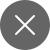# Array Data Structure and Kadane’s Algorithm

4+
##### Data Structures and algorithms are key to clearing multiple placement tests as well as for competitive programming.

A list of important data structures are listed below:

• Array
• Stacks
• Queues
• Hash tables
• Trees
• Heaps
• Graphs

## Arrays

Arrays are a collection of elements belonging to the same data type that can be easily accessed using their index value. Consider array is a pile of books on your study table (Consider 5 books) out of which one is on the desk hence you number it is 0 next as 1 next 2 and so on.

Book_prices = [101, 102, 103, 104, 105]

Consider 0, 1, 2, 3, 4 as index numbers for the books and 101, 102, 103, 104, 105 be their prices respectively. Let’s try to look at these visually.

Using the values of the indexes the element values can be accessed

For eg. Books_prices  = 104

Explanation – using the index value 3 the element i.e. the book price of index 3 can be accessed.

Arrays allow a wide range of operations that are listed below:

• Traversing – Printing all the elements of the array to their indexes
• Inserting –  To invert element in the array
• Deleting – To discard any element using their index values
• Searching – To search an index or an element in the array
• Updating – Modifying values of elements in an array

Big O Notation of all the operations are listed in the table below:

There are multiple questions to practice on various sites based on arrays, one of the most common questions in various competitive programming is finding the sum of the maximum subarray.

Find the sum of the maximum subarray

Consider an array : [1, -3, 2, 1, -1]

Sub-Arrays are a small part of an array in a continuous manner

Here the sub-arrays can be : [1, -3, 2, 1], [1, -3, 2], [1, -3] and many more…

We now will find the maximum sum that is achievable from a subarray of this array using two methods

• Brute Force Method
• The Brute Force method is an uncomplicated and simple approach to any problem that is the guaranteed way to get a solution to a problem and ideal for basic problem-solving questions.
• The brute force method compromises in terms of its time complexity and the algorithm analysis is often found to be above the O(N!) order of growth.
• Unlike the Brute force method, Kadane’s Algorithm is based on logic building as well as using the concepts of the array to the best of their use making the algorithm efficient.
• Kadane’s Algorithm is mostly used to find the maximum sum of the subarray.
• The time complexity of Kadane’s algorithm is found to be O(N).

Algorithm:

Simple!

• Traverse through each and every possible subarray using a loop
• Initialize two variables i.e. Maximum_sum and current_sum
• Find the sum of the continuous array and store them as current sum, Keep track if the current sum is greater or lesser than the max sum.
• If (Current_sum > Maximum_sum) → maximum_sum = cu
• rrent sum
• If (Current_sum < 0) → current_sum = 0
• Return final value of maximum_sum after the loop is executed

Explanation:

• The current sum and maximum sum are 0, now using the loop we check the sum of every sub-array by incrementing their index values.
• At index 0 the maximum sum is 1 which is greater than the maximum sum hence the value of the maximum sum is replaced by the current sum. But, the array is not terminated yet hence we move forward.
• Now, the index value is increment hence both 1 and -3 are considered (1+(-3) = -2) since the current value is lesser than 0, the current sum is assigned 0, and the elements 1 and -3 are finalized to be neglected.
• Moving ahead the next index i.e. index 2 is considered with the value 2 the value is greater than the current sum that is 0 hence the maximum sum is replaced with 2 i.e. the current sum.
• Again the index is incremented to index 3 with the element value as 1 here, (2+1 = 3)  which is greater than the current sum, and hence current sum and maximum sum are now 3.
• Lastly, we reach index 4 with element value -1 (2+1+(-1) = 2) which is lesser than the current sum and hence it can be neglected
• Finally, we return the maximum value that is 3!

Since we traverse through each element only once the Big O notation of Kadane’s algorithm is found to be O(N).4+

#### You may also like...